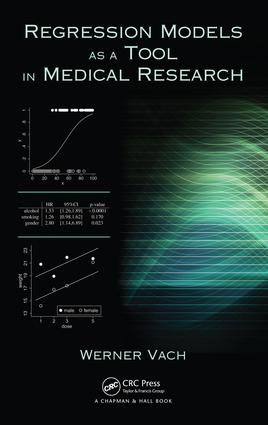# Regression Models as a Tool in Medical Research

## 1st Edition

Chapman and Hall/CRC

496 pages

##### Purchasing Options:\$ = USD
Hardback: 9781466517486
pub: 2012-11-27
\$110.00
x
eBook (VitalSource) : 9780429185526
pub: 2012-11-27
from \$28.98

FREE Standard Shipping!

### Description

While regression models have become standard tools in medical research, understanding how to properly apply the models and interpret the results is often challenging for beginners. Regression Models as a Tool in Medical Research presents the fundamental concepts and important aspects of regression models most commonly used in medical research, incl

THE BASICS: Why Use Regression Models? An Introductory Example. The Classical Multiple Regression Model. Adjusted Effects. Inference for the Classical Multiple Regression Model. Logistic Regression. Inference for the Logistic Regression Model. Categorical Covariates. Handling Ordered Categories: A First Lesson in Regression Modeling Strategies. The Cox Proportional Hazard Model. Common Pitfalls in Using Regression Models. ADVANCED TOPICS AND TECHNIQUES: Some Useful Technicalities. Comparing Regression Coefficients. Power and Sample Size. The Selection of the Sample. The Selection of Covariates. Modeling Nonlinear Effects. Transformation of Covariates. Effect Modification and Interactions. Applying Regression Models to Clustered Data. Applying Regression Models to Longitudinal Data. The Impact of Measurement Error. The Impact of Incomplete Covariate Data. RISK SCORES AND PREDICTORS: Risk Scores. Construction of Predictors. Evaluating the Predictive Performance. Outlook: Construction of Parsimonious Predictors. MISCELLANEOUS: Alternatives to Regression Modeling. Specific Regression Models. Specific Usages of Regression Models. What Is a Good Model? Final Remarks on the Role of Prespecified Models and Model Development. MATHEMATICAL DETAILS: Mathematics behind the Classical Linear Regression Model. Mathematics behind the Logistic Regression Model. The Modern Way of Inference. Mathematics for Risk Scores and Predictors. Bibliography. Index.

### About the Author

Werner Vach is a professor of medical informatics and clinical epidemiology at the University of Freiburg. Dr. Vach has co-authored more than 150 publications in medical journals. His research encompasses biostatistics methodology in the areas of incomplete covariate data, prognostic studies, diagnostic studies, and agreement studies.

### Subject Categories

##### BISAC Subject Codes/Headings:
MAT029000
MATHEMATICS / Probability & Statistics / General
MED090000
MEDICAL / Biostatistics